# Test: Electrostatic Potential & Capacitance

## 25 Questions MCQ Test Physics For JEE | Test: Electrostatic Potential & Capacitance

Description
Attempt Test: Electrostatic Potential & Capacitance | 25 questions in 30 minutes | Mock test for NEET preparation | Free important questions MCQ to study Physics For JEE for NEET Exam | Download free PDF with solutions
QUESTION: 1

### For a charged conductor of arbitrary shape, inside the conductor

Solution:

The potential difference between any two points inside the hollow conductor is zero. This means that the potential at all points inside the hollow charged conductor is the same and it is equal to the value of the potential at its surface.

The electric field on the surface of a hollow conductor is maximum and it drops to zero abruptly inside the conductor.

E = - dV/dr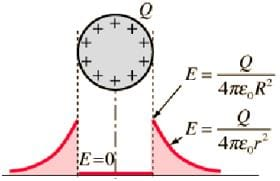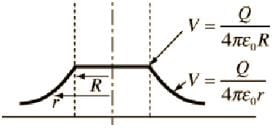QUESTION: 2

### An equipotential surface is a surface ______.

Solution:

When the potential at all points on the surface is the same, such a surface is called an equipotential surface.

The potential difference between any two points on an equipotential surface is zero.

QUESTION: 3

### A variable capacitor and an electroscope are connected in parallel to a battery. The reading of the electroscope would be decreased by_______

Solution:

An electroscope is a device that measures the potential difference.
If it is connected in parallel to the capacitor, the potential across it will be equal to the potential across the capacitor, which is equal to the potential across the battery.
On decreasing the battery potential, the potential difference across the electroscope reduces, and hence the reading reduces.

QUESTION: 4

A 2μF capacitor C1 is charged to a voltage 100 V and a 4 μF capacitor C2 is charged to a voltage 50 V. The capacitors are then connected in parallel. What is the loss of energy due to parallel connection?

Solution: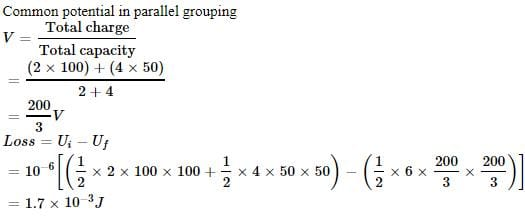QUESTION: 5

If the potential difference between the plates of a capacitor is increased by 0.1%, the energy stored in the capacitor increases by nearly:

Solution:

The intial energy stored in the capacitor C of potential difference V is
E = (1/2) CV2
When the p.d increased by ΔV, the energy stored will be ΔE.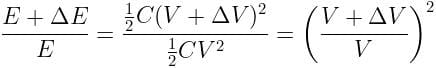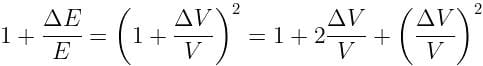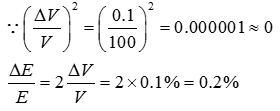QUESTION: 6

If the diameter of earth is 128 x 10km, then its capacitance will be:

Solution:

The capacitance of a spherical conductor of radius R is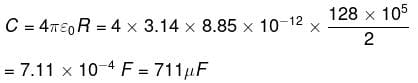QUESTION: 7

If a charge moves in an electrical field:

Solution:

When a charged particle moves in an electric field, its electric potential energy decreases, its kinetic energy will increase i.e. the total energy is conserved.

QUESTION: 8

If the charge on a capacitor is increased by 2 coulomb, the energy stored in it increases by 21%. The original charge on the capacitor (in coulomb) is:

Solution:

The initial energy of the capacitor of capacitance C and charge Q1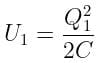When the charge increases to Q2 the change in the energy of the capacitor,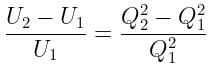Given percentage increase of energy,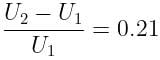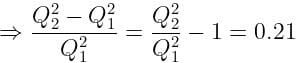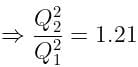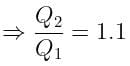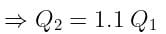Since, Q2 - Q1 = 2
⇒ Q1 = 20 C

QUESTION: 9

In series combination of capacitors, potential drops across the individual capacitors are equal to_______

Solution:

When capacitors are connected in series, they have equal charge but the potential difference across them is given by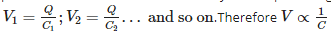QUESTION: 10

A capacitor of capacitance C is fully charged by a 200 V supply. It is then discharged through a small coil of resistance wire embedded in a thermally insulated block of specific heat 2.5×102Jkg−1K−1 and of mass 0.1 kg. If the temperature of the block rises by 0.4 K, what is the value of C?

Solution:

Energy stored in the capacitor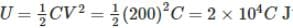This is released as heat when the capacitor discharges through the metal block. The quantity of heat = mass xs p.heatx rise in temperature.
Q = m x s x Δθ = 0.1 x 2.5 x 102 x 0.4 = 10 J
U = Q; 2 x 104 C = 10; C = 5 x 10-4 F = 500 μF

QUESTION: 11

64 water drops having equal charges combine to form one bigger drop. The capacitance of the bigger drop, as compared to that of smaller drop will be:

Solution:

Capacitance of the small drop of radius r C8 = 4πε0r  and that of the big drop of radius R is CB = 4πε0R .
The volume of 64 small drops,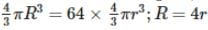The ratio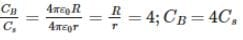QUESTION: 12

An electrolytic capacitor is marked 8 μF, 220 V. It can be used in a circuit where the p.d. across the capacitor may be:

Solution:

The breakdown of the potential of the capacitor is 220 V.

In order to prevent damage to a capacitor, it should be always used in a circuit where the p.d is less than its breakdown potential. The p.d difference can only be 200 V.

QUESTION: 13

The capacitance of a parallel plate capacitor is 5 μF. When a glass slab of thickness equal to the separation between the plates is introduced between the plates, the potential difference reduces to 1/8 of the original value. The dielectric constant of glass is

Solution:

When a dielectric of constant K is introduced between the plates of a capacitor, the potential V reduces to V/K. Therefore K=8

QUESTION: 14

For a parallel plate capacitor ______________ possible potential difference between the capacitor plates.

Solution:

When a dielectric is introduced between two charged plates of a capacitor having a charge Q and maintained at a potential difference of V, a reverse electric field is set up inside the dielectric due to dielectric polarization. This reduces the electric field in between the plates. The potential is also reduced.

Maximum potential is dependent on the charge on the plates. As the charge remains constant, the presence of the dielectric decreases the maximum potential between the plates.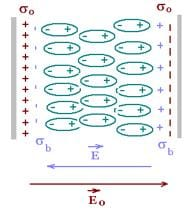QUESTION: 15

Which of the following is true about equipotential lines?

Solution:

Electric field lines show the direction of the electric field at the point. If the electric field lines were tangential, parallel, or opposite to the equipotential surface, a tangential field will exist on the surface and work done in moving a charge on the surface is not zero.

Therefore electric field lines are always perpendicular to the equipotential surface.

QUESTION: 16

In electrolytic capacitors positive terminal is ________

Solution:

Aluminium electrolytic capacitors have the Aluminium foil anode (positive terminal) which is attached and covered with a layer of Aluminium Oxide which acts as a dielectric. The whole assembly is covered using a paper separator soaked in electrolyte such as, Borax or Glycol and covered by Aluminium foil which acts as cathode ( negative electrode)

QUESTION: 17

If a positive charge moves opposite to the direction of the electric field :

Solution:

A positive charge experiences a force in the direction of the field.
If it moves opposite to the direction of the field, negative work is done by the electric field on the charge.
Potential energy of the charge increases since an external source is required to do work on the charge against the direction of the field, and the work done is stored in the charge as its potential energy.

QUESTION: 18

Submarine cables act as __________

Solution:

A submarine cable consists of an inner conductor which carries power. This conductor is covered by an insulator, which acts as a dielectric. The dielectric material is covered by a metal coating called shield, which is connected to ground.
The cable acts as a cylindrical capacitor, with the conductor acting as the inner cylinder, and the metal shield as the outer cylinder which is connected to earth.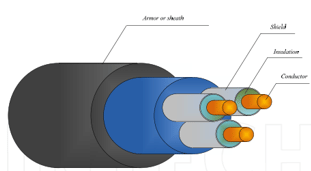QUESTION: 19

When 2 charged conductors are connected by a wire,

Solution:

Let the two conductors have capacitances C1 and C2 and let their potentials be V1 and V2 .
Total initial energy of the conductors,  U= (1/2)C1V12 + (1/2)C2V22
When two charged conductors are connected by a wire, the flow of charge stops when the potentials are equalized.
Let V be the common potential and Q1 and Q2 be the charges on the conductors after the common potential is attained.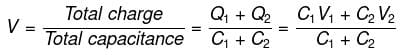The final energy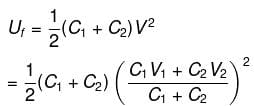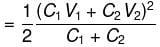Therefore,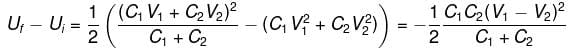QUESTION: 20

Electrostatic shielding is based on the _______

Solution:

The electric field inside a cavity is always zero. The potential inside a cavity of a conductor is constant.
The potential difference between any two points inside a charged conductor is zero. This phenomenon is called electrostatic shielding.

QUESTION: 21

Two metallic spheres of radii 1 cm and 3 cm are given charges of (-1 x 10-2 C) and 5 x 10-2 C , respectively. If these are connected by a conducting wire, the final charge on the bigger sphere is:

Solution:

Qtotal​ = 4×10−2C

Charge distribution α capacitance α radius

Qsmall​:Qbig​=1:3

Qbig​= 3/4 ×4×10−2 = 3×10−2C

QUESTION: 22

Consider the situation shown in the figure. The capacitor A has a charge q on it whereas B is uncharged. The charge appearing on the capacitor B a long time after the switch is closed is: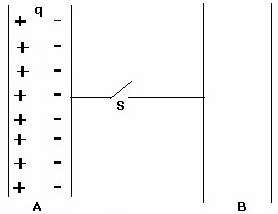Solution:

Since there is an equal number of positive and negative charges on capacitor A, the charges won't flow to capacitor B as the charge are held by strong electrostatic force.
The capacitor B will acquire a charge only if a battery (driving force) is put in the circuit.

QUESTION: 23

Three capacitors, each of capacitance C = 3 mF, are connected as shown in the figure. The equivalent capacitance between points P and S is ?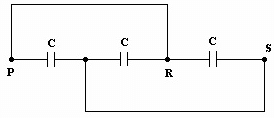Solution: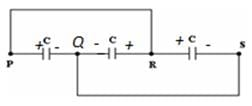If P is at positive potential, then Q is at negative potential and R is at positive potential. The system therefore reduces to 3 capacitors in parallel. C= 9μF

QUESTION: 24

When two capacitors are put in series, the equivalent capacitance is ________

Solution:

When two capacitors C1 and C2 are connected in series, the reciprocal of the equivalent capacitance in series is equal to the sum of the reciprocals of the two individual capacitances.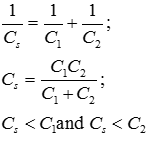It has value lesser than the least value of capacitance.

QUESTION: 25

When a capacitor is connected to a battery:

Solution:

When an uncharged capacitor is connected to a battery, charges flow from the poles of the battery to the plates of the capacitor and this process continues till the potential across the capacitor attains the potential difference of the battery. The current flow in the circuit till the time the capacitor is charged and then it ceases.Use Code STAYHOME200 and get INR 200 additional OFF Use Coupon Code